# RD Sharma Solutions for Class 9 Maths Chapter 13 Linear Equations in Two Variables Exercise 13.2

Chapter 13, exercise 13.2 helps students to solve the linear equations in an easy way. Let ax+ by + c = 0 where a,b, c are real numbers, a,b are not equal to zero, and x, y are variable, then any pair of values of x and y which satisfies the equation is called a solution of it. Learn more about linear equations and its properties in detail with complete exercise wise RD Sharma Solutions to prepare well for solving different complicated problems related to Linear Equations in Two Variables quickly.

## Download PDF of RD Sharma Solutions for Class 9 Maths Chapter 13 Linear Equations in Two Variables Exercise 13.2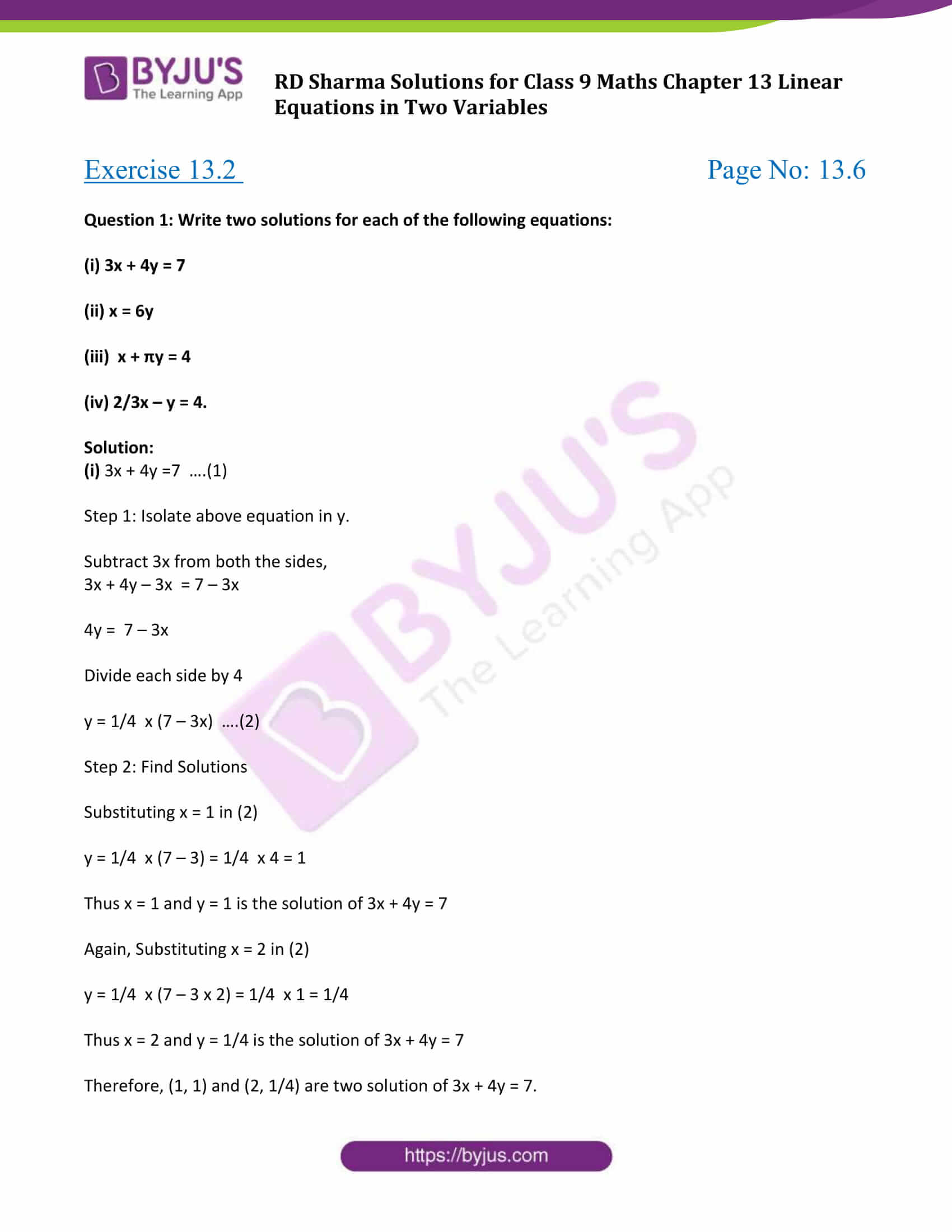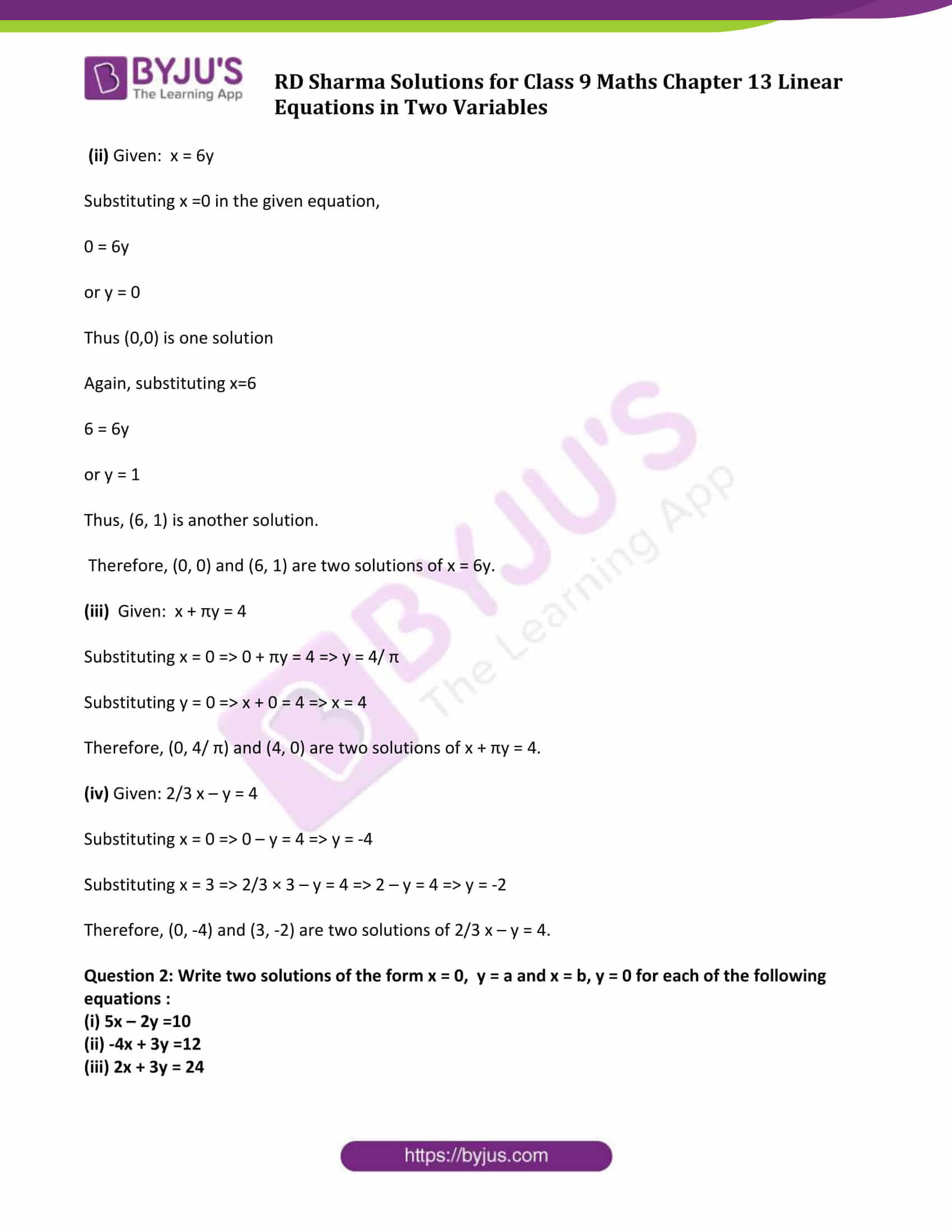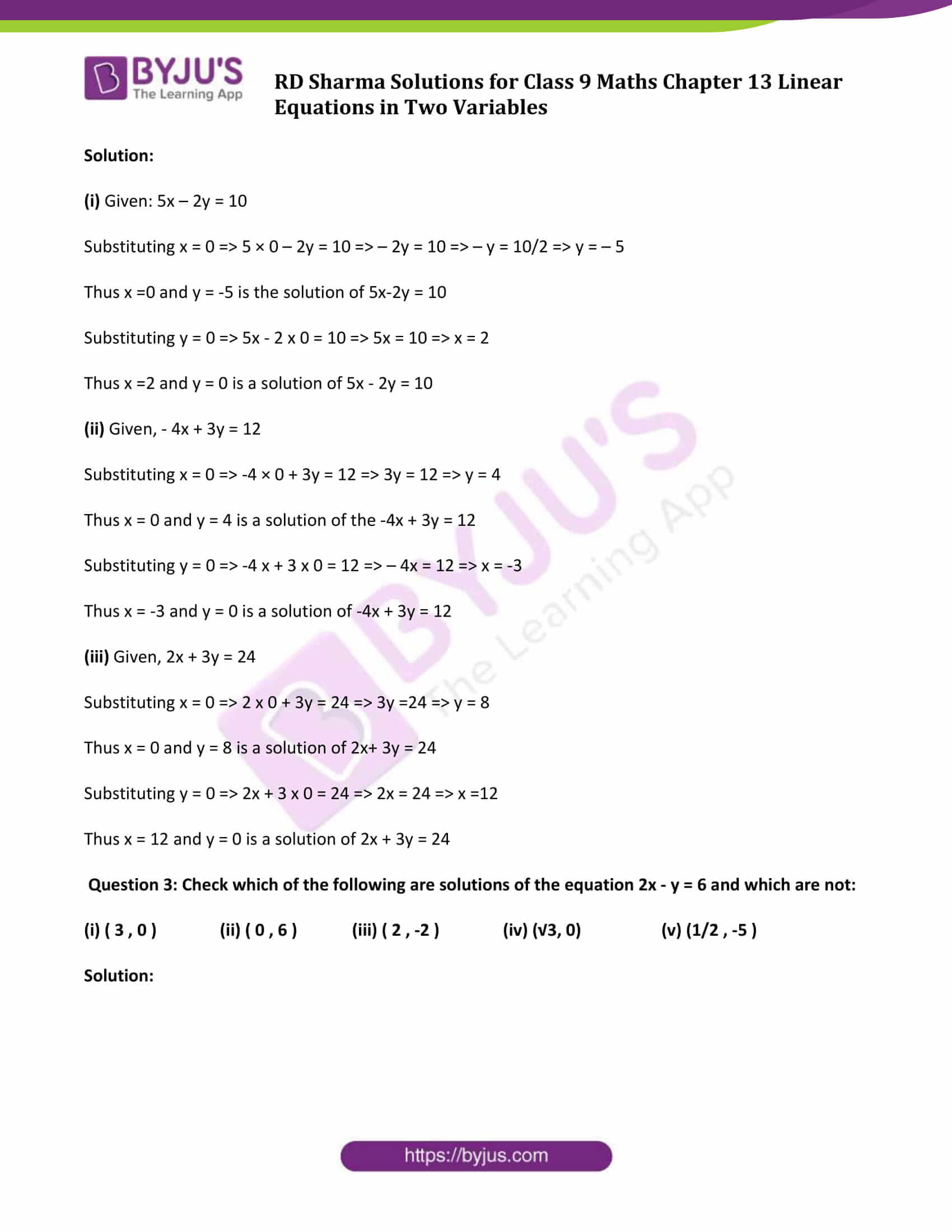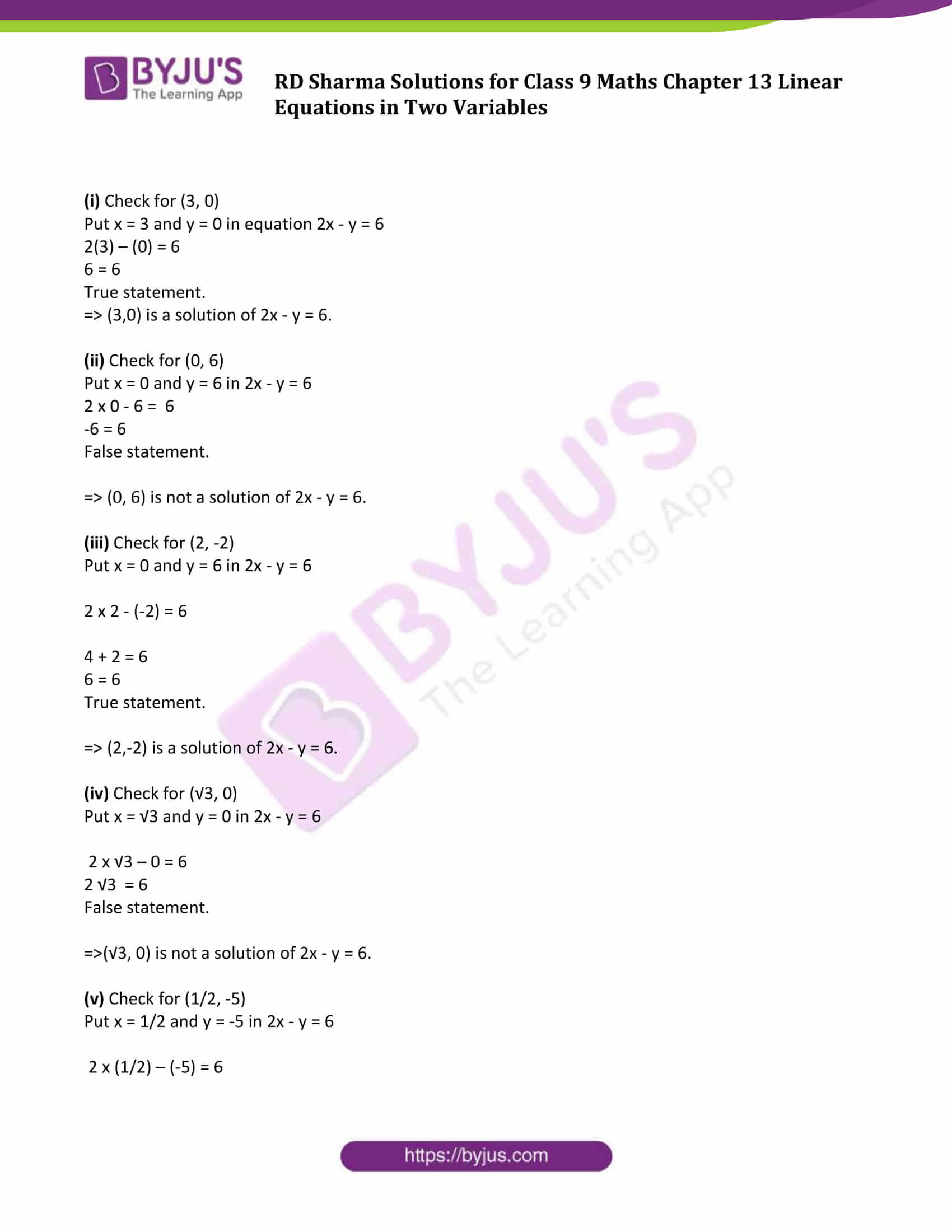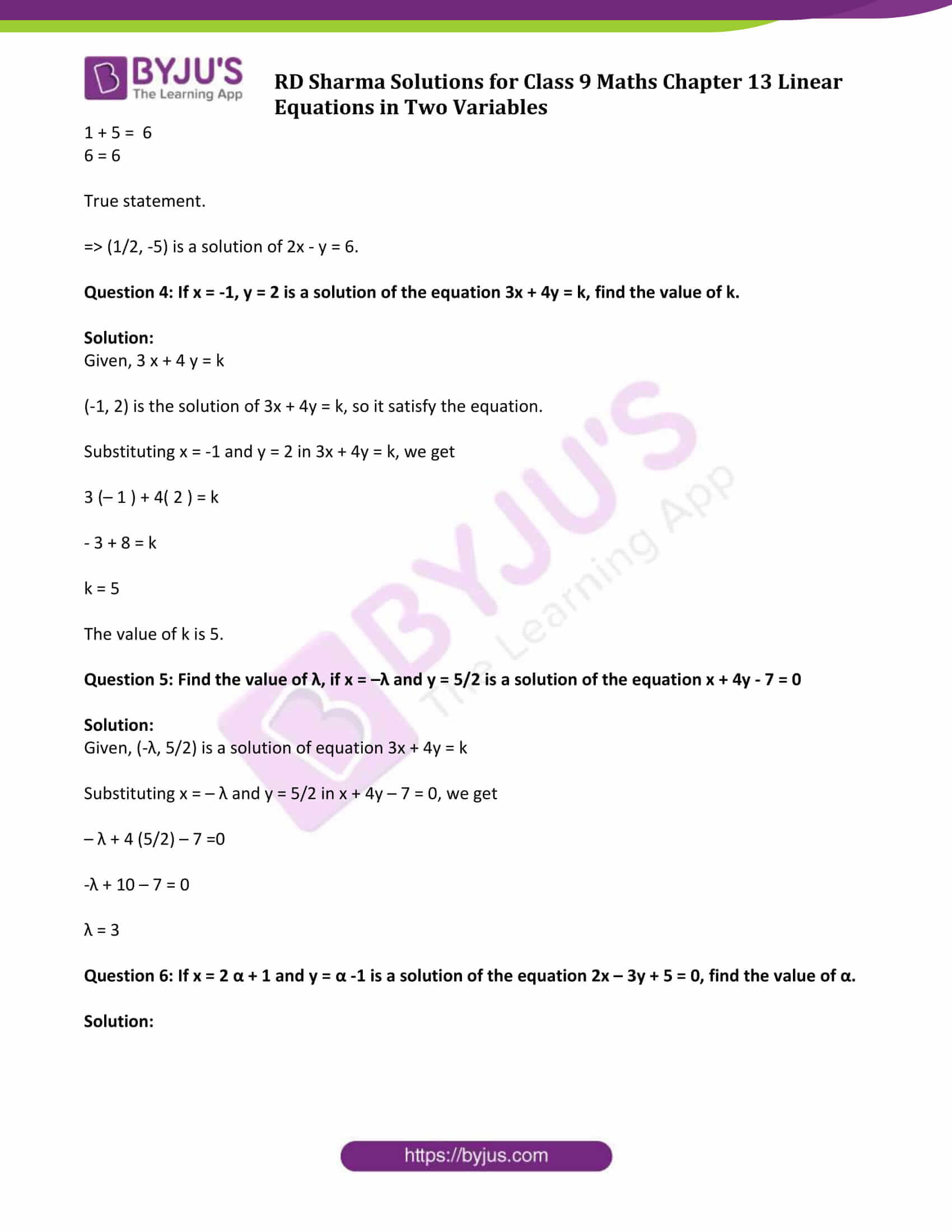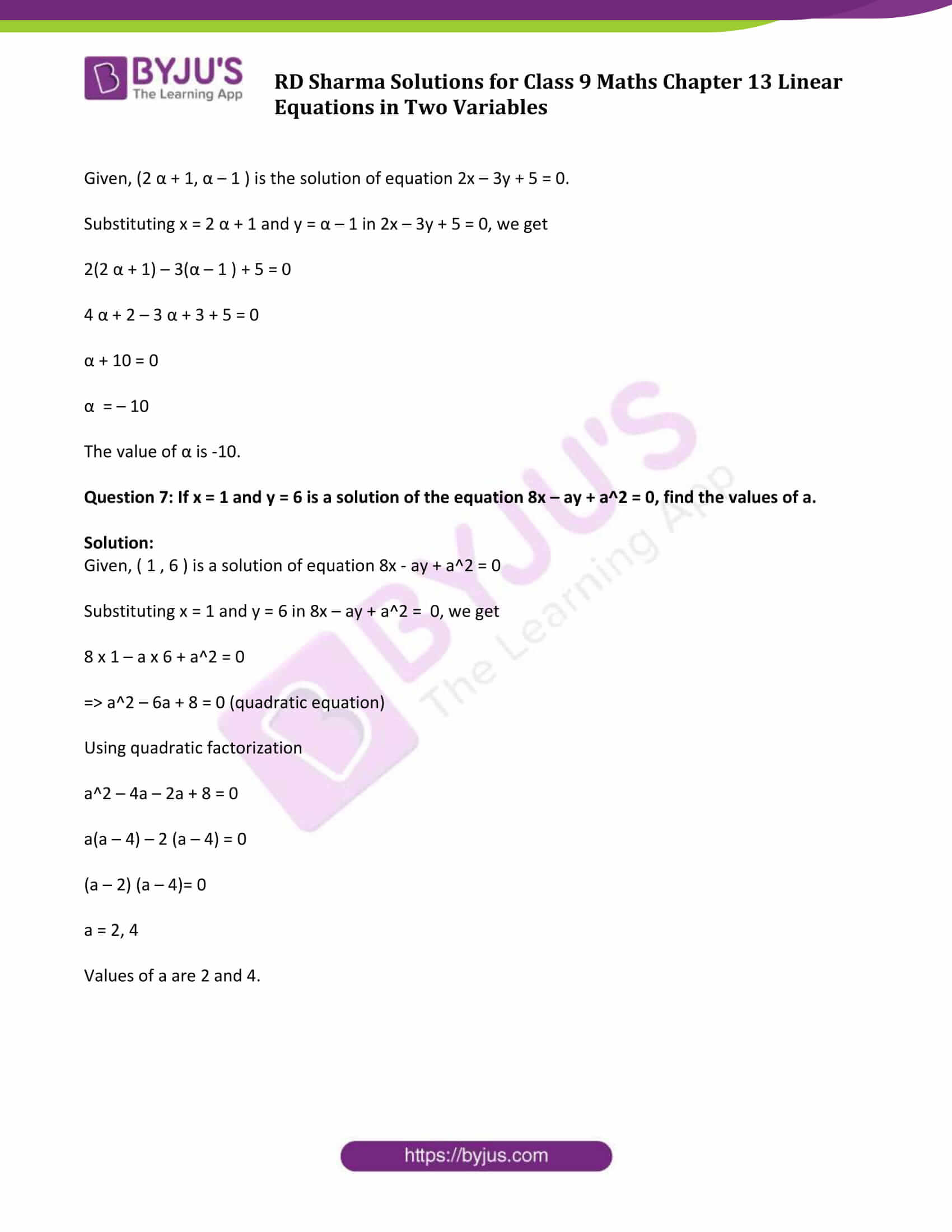### Access Answers to Maths RD Sharma Solutions for Class 9 Chapter 13 Linear Equations in Two Variables Exercise 13.2 Page number 13.6

Question 1: Write two solutions for each of the following equations:

(i) 3x + 4y = 7

(ii) x = 6y

(iii) x + πy = 4

(iv) 2/3x – y = 4.

Solution:

(i) 3x + 4y =7 ….(1)

Step 1: Isolate above equation in y.

Subtract 3x from both the sides,

3x + 4y – 3x = 7 – 3x

4y = 7 – 3x

Divide each side by 4

y = 1/4 x (7 – 3x) ….(2)

Step 2: Find Solutions

Substituting x = 1 in (2)

y = 1/4 x (7 – 3) = 1/4 x 4 = 1

Thus x = 1 and y = 1 is the solution of 3x + 4y = 7

Again, Substituting x = 2 in (2)

y = 1/4 x (7 – 3 x 2) = 1/4 x 1 = 1/4

Thus x = 2 and y = 1/4 is the solution of 3x + 4y = 7

Therefore, (1, 1) and (2, 1/4) are two solution of 3x + 4y = 7.

(ii) Given: x = 6y

Substituting x =0 in the given equation,

0 = 6y

or y = 0

Thus (0,0) is one solution

Again, substituting x=6

6 = 6y

or y = 1

Thus, (6, 1) is another solution.

Therefore, (0, 0) and (6, 1) are two solutions of x = 6y.

(iii) Given: x + πy = 4

Substituting x = 0 ⇒ 0 + πy = 4 ⇒ y = 4/ π

Substituting y = 0 ⇒ x + 0 = 4 ⇒ x = 4

Therefore, (0, 4/ π) and (4, 0) are two solutions of x + πy = 4.

(iv) Given: 2/3 x – y = 4

Substituting x = 0 ⇒ 0 – y = 4 ⇒ y = -4

Substituting x = 3 ⇒ 2/3 × 3 – y = 4 ⇒ 2 – y = 4 ⇒ y = -2

Therefore, (0, -4) and (3, -2) are two solutions of 2/3 x – y = 4.

Question 2: Write two solutions of the form x = 0, y = a and x = b, y = 0 for each of the following equations :

(i) 5x – 2y =10

(ii) -4x + 3y =12

(iii) 2x + 3y = 24

Solution:

(i) Given: 5x – 2y = 10

Substituting x = 0 ⇒ 5 × 0 – 2y = 10 ⇒ – 2y = 10 ⇒ – y = 10/2 ⇒ y = – 5

Thus x =0 and y = -5 is the solution of 5x-2y = 10

Substituting y = 0 ⇒ 5x – 2 x 0 = 10 ⇒ 5x = 10 ⇒ x = 2

Thus x =2 and y = 0 is a solution of 5x – 2y = 10

(ii) Given, – 4x + 3y = 12

Substituting x = 0 ⇒ -4 × 0 + 3y = 12 ⇒ 3y = 12 ⇒ y = 4

Thus x = 0 and y = 4 is a solution of the -4x + 3y = 12

Substituting y = 0 ⇒ -4 x + 3 x 0 = 12 ⇒ – 4x = 12 ⇒ x = -3

Thus x = -3 and y = 0 is a solution of -4x + 3y = 12

(iii) Given, 2x + 3y = 24

Substituting x = 0 ⇒ 2 x 0 + 3y = 24 ⇒ 3y =24 ⇒ y = 8

Thus x = 0 and y = 8 is a solution of 2x+ 3y = 24

Substituting y = 0 ⇒ 2x + 3 x 0 = 24 ⇒ 2x = 24 ⇒ x =12

Thus x = 12 and y = 0 is a solution of 2x + 3y = 24

Question 3: Check which of the following are solutions of the equation 2x – y = 6 and which are not:

(i) ( 3 , 0 ) (ii) ( 0 , 6 ) (iii) ( 2 , -2 ) (iv) (√3, 0) (v) (1/2 , -5 )

Solution:

(i) Check for (3, 0)

Put x = 3 and y = 0 in equation 2x – y = 6

2(3) – (0) = 6

6 = 6

True statement.

⇒ (3,0) is a solution of 2x – y = 6.

(ii) Check for (0, 6)

Put x = 0 and y = 6 in 2x – y = 6

2 x 0 – 6 = 6

-6 = 6

False statement.

⇒ (0, 6) is not a solution of 2x – y = 6.

(iii) Check for (2, -2)

Put x = 0 and y = 6 in 2x – y = 6

2 x 2 – (-2) = 6

4 + 2 = 6

6 = 6

True statement.

⇒ (2,-2) is a solution of 2x – y = 6.

(iv) Check for (√3, 0)

Put x = √3 and y = 0 in 2x – y = 6

2 x √3 – 0 = 6

2 √3 = 6

False statement.

⇒(√3, 0) is not a solution of 2x – y = 6.

(v) Check for (1/2, -5)

Put x = 1/2 and y = -5 in 2x – y = 6

2 x (1/2) – (-5) = 6

1 + 5 = 6

6 = 6

True statement.

⇒ (1/2, -5) is a solution of 2x – y = 6.

Question 4: If x = -1, y = 2 is a solution of the equation 3x + 4y = k, find the value of k.

Solution:

Given, 3 x + 4 y = k

(-1, 2) is the solution of 3x + 4y = k, so it satisfy the equation.

Substituting x = -1 and y = 2 in 3x + 4y = k, we get

3 (– 1 ) + 4( 2 ) = k

– 3 + 8 = k

k = 5

The value of k is 5.

Question 5: Find the value of λ, if x = –λ and y = 5/2 is a solution of the equation x + 4y – 7 = 0

Solution:

Given, (-λ, 5/2) is a solution of equation 3x + 4y = k

Substituting x = – λ and y = 5/2 in x + 4y – 7 = 0, we get

– λ + 4 (5/2) – 7 =0

-λ + 10 – 7 = 0

λ = 3

Question 6: If x = 2 α + 1 and y = α -1 is a solution of the equation 2x – 3y + 5 = 0, find the value of α.

Solution:

Given, (2 α + 1, α – 1 ) is the solution of equation 2x – 3y + 5 = 0.

Substituting x = 2 α + 1 and y = α – 1 in 2x – 3y + 5 = 0, we get

2(2 α + 1) – 3(α – 1 ) + 5 = 0

4 α + 2 – 3 α + 3 + 5 = 0

α + 10 = 0

α = – 10

The value of α is -10.

Question 7: If x = 1 and y = 6 is a solution of the equation 8x – ay + a2 = 0, find the values of a.

Solution:

Given, ( 1 , 6 ) is a solution of equation 8x – ay + a2 = 0

Substituting x = 1 and y = 6 in 8x – ay + a2 = 0, we get

8 x 1 – a x 6 + a2 = 0

⇒ a2 – 6a + 8 = 0 (quadratic equation)

a2 – 4a – 2a + 8 = 0

a(a – 4) – 2 (a – 4) = 0

(a – 2) (a – 4)= 0

a = 2, 4

Values of a are 2 and 4.

## RD Sharma Solutions for Class 9 Maths Chapter 13 Linear Equations in Two Variables Exercise 13.2

RD Sharma Solutions Class 9 Maths Chapter 13 Linear Equations in Two Variables Exercise 13.2 is based on finding a solution of a linear equation. Students can download RD Sharma solutions for class 9 which are prepared by subject experts and start practicing to score good marks.